# Experimental Study of Underwater Wireless Optical Communication from Clean Water to Turbid Harbor under Various Conditions

Experimental Study of Underwater Wireless Optical Communication from Clean Water to Turbid Harbor under Various Conditions

Department of Laser and Optoelectronics Engineering, University of Technology, Baghdad 10001, Iraq

Optics Techniques Department, Dijlah University College, Baghdad 10001, Iraq

Computer Techniques Engineering Department, Dijlah University College, Baghdad 10001, Iraq

Corresponding Author Email:
salahaldeen.a.taha@uotechnology.edu.iq
Page:
219-226
|
DOI:
https://doi.org/10.18280/ijdne.160212
25 November 2020
|
Accepted:
22 January 2021
|
Published:
30 April 2021
| Citation

OPEN ACCESS

Abstract:

In this paper, texts were experimentally transmitted by pulse width modulation (PWM) using an underwater wireless optical communication system (UWOC) in a channel containing water of varying salinity as a result of changes in the concentration of sodium chloride (NaCl). Mathematical equations are used using a MATLAB program to compare theoretical and practical results at different slop angle (θ0). (NaCl) concentration was changed from (0% w/v) to (90% w/v) to achieve different salinity of water (i.e., from clear water to turbid water). A diode laser with a power of 30 mW and a wavelength of 532 nm has been employed in the transmitter. The experimental results show that the extinction coefﬁcient or the overall attenuation C(λ) is equal to (0.083/m) in the water containing a low concentration of (NaCl) which is consistent with pure seawater. Additionally, the obtained optical power (PR) and the signal to noise ratio (S/N) decreases to (27.6) mW and (23.99) dB, respectively. Furthermore, it was found that the water had a maximum total attenuation C(λ) equal to (2.565/m) in the water containing a high concentration of (NaCl) which was compatible with turbid harbour water, as well as the received power and (S/N) decreases to (2.306) mW and (13.2) dB, respectively. The theoretical results were similar to the practical results when the slope angle of the target or detector relative to the optical transmitter was (zero).

Keywords:

UWOC, slope angle, line of sight transmission (LOS), extinction coefﬁcient, signal to noise ratio (S/N)

1. Introduction

Underwater optical communication has become dominant in the telecommunications world due to the large bandwidth, response speed and security. Underwater wireless communication is possible through acoustic waves, radio frequency (RF) waves and optical waves. The bandwidth of light waves is greater than that of acoustic waves and radio waves (RF), so more information can be sent in less time . According to the US Oceanic and Atmospheric Administration Statistics, 97% of the Earth's area is covered by ocean waters .

Today, underwater communication is carried out using acoustic waves, RF waves and optical waves. Acoustic communication is the most common technology used underwater and over long distances. However, Acoustic waves have many disadvantages such as scattering, high attenuation, low propagation velocity and negative effects on underwater mammals [3, 4]. To eliminate this channel problem (UAWC) the researchers used low frequency (RF) waves and microwaves for underwater radio communication and were able to transmit data for tens of kilometers [5, 6]. Data was transmitted using microwaves in underwater wireless communications for a horizontal distance of 85 m and 90 m at a data rate of 500 Kbps. In other studies, the data rate is 100 Mbps for a distance of 100 meters. RF and microwaves suffer from high attenuation.

The attenuation in Ocean is about 169 dB/m for the 2.4 kHz band, while the freshwater attenuation is higher, which is 189 dB /m. Underwater RF work requires large antennas. At lower frequencies, the attenuation decreases against a lower data transmission rate . Due to the limitation of the bandwidth and low data rate in acoustic waves and RF waves for underwater wireless communication, an alternative underwater transmitter system, (UWOC) was used, which provides high speed and large data transmission rate, scalability, reliability, and flexibility [5, 8]. Optical communication relative to acoustic communication and RF waves has a higher bandwidth, better security and lower response time, so optical communication has been used photography and video transmission in real-time . However, despite absorption, dispersion and disturbance, optical communications remain suitable for short-range communications of less than 100 meters [10-13].

Optical communications have many defects such as absorption, scattering, and disturbances, which lead to weak signal and limit the range to less than 100 meters [14, 15]. Optical disturbances in water are due to random changes such as temperature difference and salinity changes [16, 17] but optical disturbances in the atmosphere due to changes in pressure and temperature [18, 19]. In addition to the attenuation in UWOC channel due to changes in temperature and salinity, the air bubbles cause random disturbances, which leads to attenuation of the optical signal . Attenuation also occurs when the diver sends optical signals due to the air bubbles that come out from the diver .

The random fluctuations of the optical signal due to the air bubbles were studied in the laboratory . The density of the statistical distribution of the air bubbles was studied and predicted. The performance of the UWOC system was analyzed by estimating the density of the air bubbles. Bernotas and Charles  and Oubei et al.  presented the statistical distribution in the laboratory of the temperature gradient and its effect on optical disturbances that lead to attenuation in the optical signal. Comprehensive studies were done on the UWOC channel, and it was found that the light propagation within the optical channel is affected by 3 main factors: absorption, scattering and turbulence .

When the light wave passes through the UWOC channel, it became clear that the photons interact with water molecules and particles, and in this case the photon energy will be dissipated thermally and this is called the absorption process and is expressed by the absorption coefficient α(λ) mathematically , (λ) is the wavelength of the light used. Moreover to the change in the direction of a portion of the photons as a result of colliding with different objects in the water, some photons will be captured in the receiver, this is called the scattering process, which is represented by the scattering coefficient β(λ), in addition the scattering of photons temporarily leads to diffuse and delay the optical signal, which leads to the determination of the data transmission rate in the UWOC channel. The loss of light energy in the UWOC channel is described by the extinction coefficient, which is C(λ) .

In the past few years, many valuable researches have been carried out, such as designing a reliable and intelligent UWOC channel to reduce the attenuation of the optical signal using multi-input and multi-output (MIMO) [27, 28] using Monte Carlo (MC) numerical simulations . The serial and multi-hop relay reduced the attenuation in the channel and showed a greater contact range from one point to another [30, 31].

The turbulence in water due to an increase in salinity or the presence of air bubbles leads to an increase in the interaction between optical photons and particles and objects in the water, which leads to increased absorption and scattering and increased losses, and this also depends on the type of water and the strength of the turbulence. For example, in turbid waters, the frequent scattering is very large, which reduces the transmission range to 10 meters .

The sharp and transmitting laser beam will propagate over a large spatial area at the level of the receiver. Therefore, the received signal pattern which contains attenuation, scattering, and propagation delay in the spatial and temporal domain is similar to the LED pattern when transmitting . This means that in such type of water in the (UWOP) channel it is possible to use the sharp laser beam or the LED beam because the pattern will be similar in the receiver and this limits the use of beam-collimator (BC) in such conditions . On the other hand, some researchers have created and analyzed the performance of an underwater cellular wireless network based on multiple access technology or optical code division multiple access (OCDMA) [14, 20, 31].

Optical disturbances in the UWOC are the main cause of fading optical signal propagation in turbulent seawater. Optical disturbances in water are the result of random changes in the refractive index, random changes in water due to random changes in salinity and temperature of water. A lot of research has been done in describing and calculating fade statistics [32, 33]. The power spectrum has been derived for the random disturbance (UWOC) channel. The random disturbance in (UWOC) channel is due to random changes of refractive index.

Based on the power spectrum, the scintillation coefficient of the optical signal propagating in the turbulent medium was calculated. The optical signal temporal statistics was achieved in a moving aqueous medium with weak turbulence. Then, the scintillation factor was formulated for the ray propagating in the weak turbulent medium. And if we take into account the logarithmic distribution of the changes in the light intensity, the rate (BER) was calculated, for (MISO) system of (UWOC) channel, the (BER) and brightness factor have been analyzed too [34, 35].

In this research, a new method has been implemented to find out the effect of increasing the angle of inclination on the rate of transmitted data by analyzing the performance of some parameters such as received optical power (PR), the amount of signal to noise ratio (S/N) and the extinction coefﬁcient C(λ) in the receiver. The parameters were extracting practically and theoretically and comparing them when increasing or changing the angle of inclination to find out the value of the angles that lead to increase losses in the received data rate and to improve the performance of (UWOC) system.

2. Water Types and Effects in UWOC Channel

The classification of ocean water depends on the percentage of disturbances due to the concentration of dissolved salts and the rate of light affected by the phenomenon of the absorption and the scattering. Oceanic waters are classified into:

1. Pure sea water: It consists of pure water molecules (H2O) and dissolved salts (NaCl, MgCl, Na2SO, KCl, etc.), which determine the total absorption in pure sea water as the dispersion coefficient is neglected in pure sea water. In this type of water, the light beam is spread in a straight line with limited scattering.
2. Coastal ocean waters: These waters contain a high concentration of salts and dissolved particles and thus suffer from more intense absorption and dispersion.
3. Turbid harbor water: In these waters the most hostile levels of absorption and dispersion appear due to the very high concentration of suspended and solute particles. Which are also restricted to optical waves diffusion due to dissolved and suspended matter [5, 22, 23].
3. Optical Signal Fading Due to Salinity of UWOC Channel

In addition to random disturbances due to air bubbles and temperatures, there are strong random disturbances due to salinity of the water causing attenuation in the UWOC channel. It can be expected that the salinity of the water will cause more random disturbances in a channel UWOC as the random disturbances of air bubbles and temperature changes .

A new term is channel coherence time which is considered as a metric describing channel fading coefficient. Channel coherence time represents the average time in which the coefficient of channel fading is constant. When the salinity in the water increases, the coherence time of the UWOC channel will be shortened, which leads to an increase in the channel fading coefficient [19, 37].

The aqueous medium contains about 80 different elements dissolved or suspended in pure water with different concentrations and various dissolved salts, the most important of which are Sodium chloride (NaCl), which absorbs light at specific wavelengths and causes scattering. It has been observed that the scattering and absorption of optical waves is reduced in the blue-green region, In the range (300-700) nm of turbid water [38, 39].

There are several types of communication link models like Line of sight communication (LOS) where the line of sight communication link between the transmitter and the receiver is a straight line and is free of obstructions. The extinction coefficient C(λ) of the aqueous medium consists of the absorption coefficient and the scattering α(λ), β(λ) respectively [40, 41].

$C(\lambda)=\beta(\lambda)+\alpha(\lambda)$     (1)

The propagation loss factor as a function of wavelength and channel length according to Berr-lambert law is [3, 42].

$L_{\mathrm{Pr}}(\lambda, z)=\exp (-C(\lambda) d)=\frac{P_{R}(\lambda)}{P_{T}(\lambda)}$     (2)

So,

$C(\lambda)=\frac{1}{d} \ln \frac{P_{T}(\lambda)}{P_{R}(\lambda)}$     (3)

where,

PT (λ): optical transmitting power;

d: The length of the water channel.

The line of sight (LOS) method has been used between the transmitter and the receiver, so theoretical received optical power equation can be used :

$P_{R}=P_{T} \eta_{T} \eta_{R}\left(e^{-\frac{C(\lambda) d}{\cos \theta}}\right)\frac{A_{R e c} \cos \theta}{\pi\left(d \tan \theta_{o}\right)^{2}}$     (4)

When the divergence angle (θ0) of the transmitter beam is very narrow (0.0344o), $\theta_{o}<<\frac{\pi}{20}$.

where,

$\eta_{T}$: Optical efficiency of the transmitter.

$\eta_{R}$: Optical efficiency of the receiver.

θ: is the angle between the perpendicular to the Rx plane and the Tx-Rx trajectory (slop angle).

θ0: The divergence angle of the transmitter beam laser.

The practical signal-to-noise ratio S/N can be calculated by the following rule .

$\mathrm{SNR}=10 \log _{10} \frac{P_{R}}{P_{n}}$     (5)

Pn: Average noise power.

The extinction coefficient C(λ) values for different types of water are indicated as follows (0.15, 0.3, and 2.19) m-1 for clean ocean water, coastal ocean water and turbid harbor water respectively .

The measured refractive index of water using Spectrophotometer is directly proportional to the Concentration of NaCl and according to the refractive index Eq. (3).

$n=\frac{C}{v}$     (6)

4. Experimental Details

4.1 Experimental procedure

The current experimental study is following the steps below:

1. By extract experimentally the performance of the parameters such as received optical power (PR), the amount of signal to noise ratio (S/N) and the extinction coefﬁcient C(λ) in the receiver depending on the line of sight transmission method (LOS) when the slope angle (θ0) between the transmitter and the receiver equal to (0o) and simulate the results using Matlab program.
2. The comparison between the practical and theoretical results by simulate the results using Matlab program to performance analysis for the same parameters above, depending on the (LOS) method when the slope angle (θ0) Between the transmitter and the receiver equal to (0o).
3.  Extraction the theoretical analysis of the performance using Matlab program for the same parameters above, depending on the (LOS) method and a comparison between them when increase the slope angle (θ0) between the transmitter and the receiver from (0o) to (30o).

4.2 Experimental setup

From the value of the total attenuation C(λ) in the water, we can know the type of water where the attenuation value C(λ) equals to (0.15, 0.3, 2.19) m-1 which is equivalent to (clean ocean, coastal ocean, turbid harbour) respectively , to obtain different values of attenuation the amount of sodium chloride NaCl was changed from (0 - 54) g, which is equivalent to the salinity ratio (0-90) % w/v respectively and each time the total attenuation value was calculated according to Eq. (3) as in figures below.

Due to decreased scattering and absorption of optical waves in the blue-green region within (300-700) nanometers for turbid water, the laser source of 532 nm wavelength has been used in our research. Practically the slope angle (θ) between the transmitter and the receiver is (zero).

The schematic diagram of the experimental setup of the underwater wireless optical communication system (UWOC) using pulse width modulation (PWM) for diode laser wavelength 532 nm is shown in Figure 1.

The texts are written by the computer and sent as a digital signal to the Arduino (DAC) in the transmitter, which regulates the pulse width according to the given program, as each character has a different pulse width from the other.

## 1.png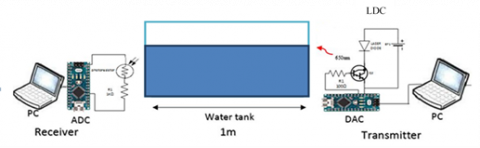Figure 1. The schematic diagram of (UWOC)

The Arduino device converts the digital signal into analog and sends it to the laser driver circuit (LDC) that provide high-speed switching and supplies with the receiver synchronization transmitted signal for each pulse. (LDC) is consist of power supply, laser diode of a wavelength 532 nm, Darlington transistor (BDx35), then the electrical analog signals that represent the transmitted information are converted into the optical signals by laser diode and sent through the water channel.

The optical signals will suffer from different loses. This information is detected by the photoresistor (LDR) in the receiver, which converts the received optical signal into an analog electrical signal, and then enters the Arduino device (ADC), which converts it into digital signals and displays the information on the computer screen. Figure 2 shows the experimental setup for the research.

## 2.png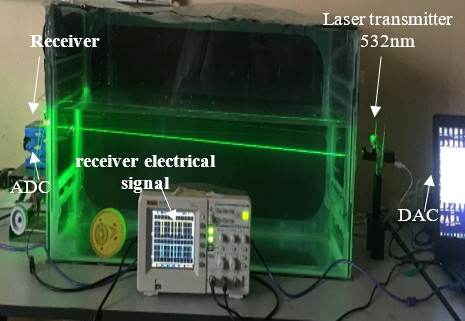Figure 2. The experimental setup for the research

5. Results and Discussion

The measured refractive index of water using Spectrophotometer is directly proportional to the Concentration of NaCl and according to the refractive index equation as shown in Eq. (6). As the concentration of NaCl in water increases the velocity of optical waves decreases. Figure 3 shows the relationship between the measured refractive index and the concentration of NaCl in water.

## 3.png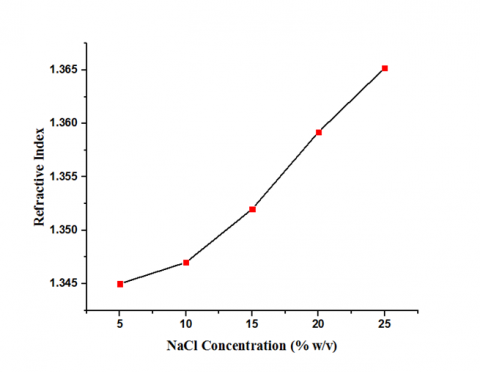Figure 3. Refractive index vs NaCl concentration

In the experimental setup the slop angle value (θ) is (zero). The power of the laser in the transmitter is 30mW, As shown in Figures 4, 5, 6, when the salt concentration is equal to zero, the water channel is similar to pure sea water and it consists of pure water molecules (H2O) and some particles suspended in it, which determine the total absorption in pure sea water, where the scattering coefficient is neglected in this type of water, so the beam of light is propagate in a straight line with limited scattering. The extinction coefﬁcient C(λ) is slightly increased due to the absorption of α(λ) and very little value of scattering β(λ) of the laser beam through the water channel, according to Eq. (1) . When the NaCl concentration becomes 1% (w/v) the water channel looks like pure sea water because the salinity of the water is low, so the value of the refractive index is less than (1), as shown in Figure 3, the received experimental power of the laser source decreased to 26mW due to absorption and low scattering along the water channel, the experimental value of (SNR) decreased to (23.8dB),the practical extinction coefﬁcient or the overall attenuation C(λ) increased to (0.15 / m) as shown in Figure 6. The increase in C(λ) occurs for two reasons:

The first: The increase in absorption losses occurs due to the photons interact with water molecules and particles, and in this case, the photon energy will be dissipated thermally which leads to a decrease in received power as shown in Figure 4, this is called the absorption process, which is represented by the absorption coefficient α(λ) .

## 4.png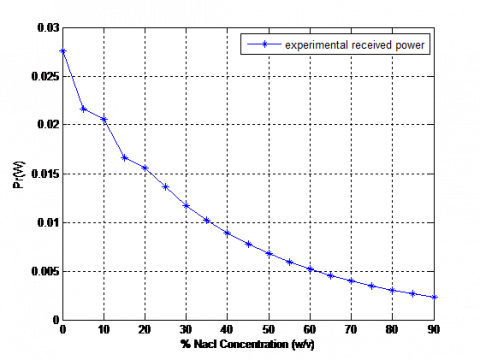Figure 4. Received power VS (NaCl) concentration at slop angle = 0o

## 5.pngFigure 5. Signal to noise ratio VS (NaCl) concentration at slop angle = 0°

## 6.png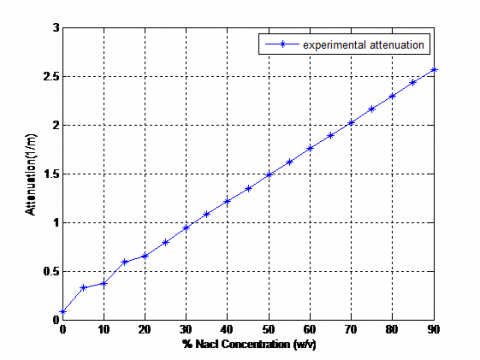Figure 6. Attenuation VS (NaCl) concentration at slop angle = 0°

The second: The little increase in scattering losses occurs because of the small increase in the concentration of NaCl that leads to change in the direction of a portion of the photons as a result of colliding with different objects in the water, some photons will be captured in the receiver, in addition the scattering of photons temporarily leads to diffuse and delay the optical signal, which leads to the determination of the data transmission rate in the UWOC channel, so this is why the (SNR) decreases as shown in Figure 5, this is called the scattering process, which is represented by the scattering coefficient β(λ) .

From Figures 4, 5, 6, When increasing the concentration of (NaCl) to 8% (w/v), The overall attenuation increases to (C(λ)=0.3/m), which is equal to the value of the attenuation in Coastal ocean waters , Which causes to increase the refractive index, that leads to decrease in the speed of light waves inside the water channel, the increase in salinity led to an increase in optical disturbances in addition to the fading of the optical signal spread in turbulent water. The random changes in the water are due to changes in the refractive index [32, 33] which led to a decrease in (SNR) to (22.8dB) and decrease the value of received power to (21mW). The increase in C(λ) is due to the large increase in absorption losses and scattering losses as a result of the collision of large number of photons with water molecules and particles that leads to change in the direction of a portion of the photons and photons energy will be dissipated thermally.

When the concentration of (NaCl) is increased to 73% (w / v), the received experimental power decreases to 4mW and the practical (SNR) value decreases to (15.7dB), which means that the signal level appeared to decrease when the concentration of (NaCl) increased and the value of practical overall attenuation C(λ) increased to (2.19 / m), which is equivalent to the overall attenuation in turbid harbor water, the increase in C(λ) is due to the large increase in absorption losses and scattering losses as a result of the collision of large number of photons with water molecules and particles that leads to change in the direction of a portion of the photons and photons energy will be dissipated thermally and limits the optical propagation due to dissolved and suspended matters , the refractive index increases to more than (1.5) as in Figure 6. In these waters the most hostile levels of absorption and dispersion appear due to the very high concentration of suspended and solute particles. Which are also restricted to optical waves diffusion due to dissolved and suspended matter [5, 44, 45].

A comparison was made between the results in two cases:

The first case: is a comparison between theoretical and practical results, when the slop angle (θ) is equal to (zero) degree between the transmitter and the receiver.

To calculate the theoretical optical power received in underwater optical communications in the case of (LOS). An Eq. (4) is used which takes into account transmitter power, telescope gain and losses.

Where we see in Figure 7 when the salinity concentration is low, the laboratory results of power is less than theoretical due to different conditions inside the laboratory causes more absorption and scattering losses. The rate of change of the theoretical and practical power is similar, and they move closer together as the salinity increases in the water, which indicates that the practical results were correct. In the (SNR) curve of Figure 8, equation No. 5 was used for theoretical results. The same rate of change occurs for theoretical and practical results, with very little difference from beginning to end due to the readings of some practical devices.

In the curve C(λ) of Figure 9, equation No. 3 was used for theoretical results. The same rate of change occurs for theoretical and practical results, with very little difference from beginning to end due to the readings of some practical devices.

## 7.png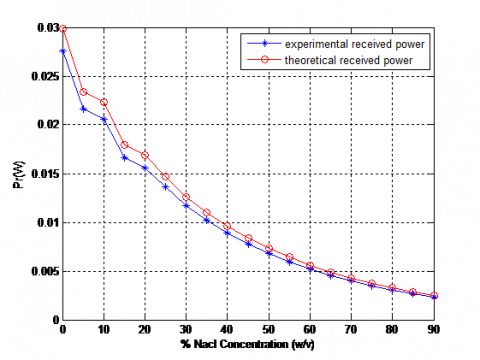Figure 7. Received power VS (NaCl) concentration at slop angle = 0°

## 8.png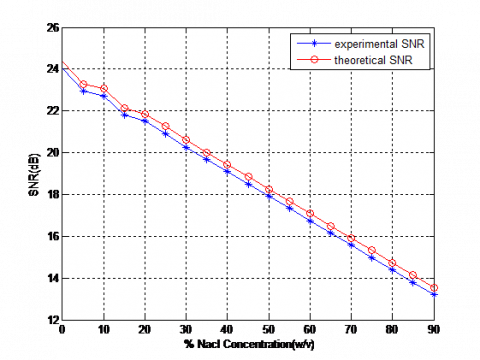Figure 8. Signal to noise ratio VS (NaCl) concentration at slop angle = 0°

## 9.pngFigure 9. Attenuation VS (NaCl) concentration at slop angle = 0o

## 10.pngFigure 10. Theoretical Received power VS (NaCl) concentration at slop angle = 0o, 30o

In the second case: a comparison was made between theoretical results only when increasing the slop angle (θ) from zero to 30 degrees to find out the effect of this increase on the received power (Pr), (SNR) and the overall attenuation C(λ) by using (UWOC) channel and (LOS) system. As above, equation No. 4 was used. From Figure 10, they show the received power in the case of the slop angle equal to 30 degrees less than it is in the case of the angle equal to zero, meaning that the greater the angle of inclination between the transmitter and the receiver, the less power in the receiver. This increase in the degree of inclination occurs when the target is moving in different directions, which leads to misalignment of the transmitted beam, so a close and stable connection must be maintained between the optical transmitters and receivers .

As above, equation No. 4 was used. From Figure 10, they show the received power in the case of the inclination angle equal to 30 degrees less than it is in the case of the angle equal to zero, which means the greater the angle of inclination between the transmitter and the receiver, the less power in the receiver. This increase in the degree of inclination occurs when the target is moving in different directions, which leads to a lack of alignment of the transmitted ray, so a close and stable connection must be maintained between the optical transmitters and receivers .

## 11.png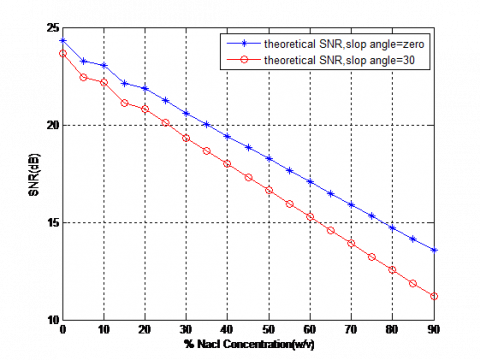Figure 11. Theoretical signal to noise ratio VS (NaCl) concentration at slop angle = 0o, 30o

## 12.png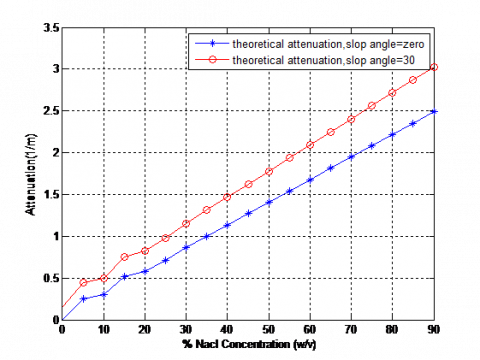Figure 12. Theoretical attenuation VS (NaCl) concentration at slop angle = 0o, 30o

In Figure 11, we see that (SNR) in the case of the angle of inclination is equal to 30 degrees less than it's in the case of the angle equal to zero. This means that the greater the angle of inclination between the sender and the receiver, the lower the rate of information sent to the target.

In Figure 12 we see that C(λ) in the case of the angle of inclination is 30 degrees greater than it is in the case of the angle equal to zero, this means the greater the angle of inclination between the transmitter and the receiver, the greater the total attenuation, and this is due to the decrease in the arrival of the optical power and the decrease (SNR) to the target, which leads to an increase in the total attenuation.

6. Conclusions

The above results indicate that the effect of (NaCl) is significant in all types of water in the world, as its increase leads to an increase in the value of attenuation for the optical signals in the (UWOC) system, which leads to decrease in the signal to noise ratio (S/N) and the received optical power as in Figures 4, 5, 6 in this paper, the (LOS) system was adopted, A comparison was made between the results in two cases:

The first case is a comparison between theoretical and practical results, when the slop angle (θ) is equal to (zero) degree between the transmitter and the receiver. The large similarity in the rate of change between theoretical and practical results was found, which means the validity of the extracted results as shown in the Figures 7, 8, 9.

In the second case, a comparison was made between the theoretical results only, when increasing the angle of inclination between the transmitter and the receiver to 30 degrees, a large overall attenuation C(λ) was observed, a decrease in the parameter of the received data (SNR) and a decrease in the optical power (pr), and this increase in the angle of inclination occurs during the movement and change of the target's direction under the water, which causes misalignment of the light beam which leads to large losses as shown in the Figures 10, 11, 12.

References

 Adnan, S.A., Ali, M.A., Sadeq, M., Kadhim, A.C., Riaz, M. (2017). Investigating link budget of underwater wireless optical communication with intensity modulation direct detection technique. in Light, Energy and the Environment, OSA Technical Digest (online) (Optical Society of America, 2017), paper JW5A.13. https://doi.org/10.1364/PV.2017.JW5A.13

 NOAA. (2017). How much water is in the ocean? https://oceanservice.noaa.gov/facts/oceanwater.html, accessed on Jan 1, 2021.

 Kumar, P.V., Praneeth, S.S., Narender, R.B. (2011). Analysis of optical wireless communication for underwater wireless communication. In International Journal of Scientific and Engineering Research, 2(6): 1-9.

 Tang, S.J., Dong, Y.H., Zhang, X.D. (2014). Impulse response modeling for underwater wireless optical communication links. in IEEE Transactions on Communications, 62(1): 226-234. https://doi.org/10.1109/TCOMM.2013.120713.130199

 Saeed, N., Celik, A., Al-Naffouri, T.Y., Alouini, M.S. (2019). Underwater optical wireless communications, networking and localization: A survey. In Ad Hoc Networks, 49: 101935 https://doi.org/10.1016/j.adhoc.2019.101935

 Adnan, S.A., Ali, M.A., Kadhim, A.C., Sadeq, M., Riaz, M. (2017). Investigating the performance of underwater wireless optical communication with intensity modulation direct detection technique. In Light Energy Environ. OSA, Washington, D.C., p JW5A.14. https://doi.org/10.1364/PV.2017.JW5A.14

 Zeng, Z., Fu, S., Zhang, H., Dong, Y., Cheng, J. (2017). A survey of underwater optical wireless communications. In IEEE Communications Surveys & Tutorials, 19(1): 204-238. https://doi.org/10.1109/COMST.2016.2618841

 Arnon, S. (2010). Underwater optical wireless communication network. In Optical Engineering, 49(1): 015001. https://doi.org/10.1117/1.3280288

 Kaushal, H., Kaddoum, G. (2016). Underwater optical wireless communication. In IEEE Access, 4: 1518-1547. https://doi.org/10.1109/ACCESS.2016.2552538

 Mital, M.E.G., Michael, Olarte, V.C., Ong, N.B.S., Ortega, D.C.F., Uy, M.T.R., Rocamora, J.M.B., Roxas, E.A., Cruz, A.R.D., Vicerra, R.R.P. (2017). Characterization of underwater optical data transmission parameters under varying conditions of turbidity and water movement. In 2017 5th International Conference on Information and Communication Technology, pp. 1-6. https://doi.org/10.1109/ICoICT.2017.8074642

 Gabriel, C., Khalighi, M.A., Bourennane, S., Léon, P., Rigaud, V. (2013). Monte-Carlo-based channel characterization for underwater optical communication systems. Journal of Optical Communications and Networking, 5(1): 1. https://doi.org/10.1364/JOCN.5.000001

 Cox, W.C. (2012). Simulation, modeling, and design of underwater optical communication systems. Ph.D. thesis. http://www.lib.ncsu.edu/resolver/1840.16/7588

 Mobley, C.D., Preisendorfer, R.W. (1994). Light and Water: Radiative Transfer in Natural Waters. Academic Press.

 Akhoundi, F., Jamali, M.V., Hassan, N.B., Beyranvand, H., Minoofar, A., Salehi, J.A. (2016). Cellular underwater wireless optical CDMA network: Potentials and challenges. IEEE Access, 4: 4254-4268. https://doi.org/10.1109/ACCESS.2016.2593398

 Geldard, C.T., Thompson, J., Popoola, W.O. (2019). An overview of underwater optical wireless channel modelling techniques: (Invited Paper). in 2019 International Symposium on Electronics and Smart Devices, pp. 1-4. https://doi.org/10.1109/ISESD.2019.8909494

 Korotkova, O., Farwell, N., Shchepakina, E. (2012). Light scintillation in oceanic turbulence. In Waves in Random and Complex Media, 22(2): 260-266. https://doi.org/10.1080/17455030.2012.656731

 Thorpe, S.A. (2012). An introduction to Ocean Turbulence. Cambridge University Press. https://doi.org/10.1017/CBO9780511801198

 Jazayerifar, M., Salehi, J.A. (2006). Atmospheric optical CDMA communication systems via optical orthogonal codes. In IEEE Transactions on Communications, 54(9): 1614-1623. https://doi.org/10.1109/TCOMM.2006.881245

 Jamali, M.V., Mirani, A., Parsay, A., Abolhassani, B., Nabavi, P., Chizari, A., Khorramshahi, P., Abdollahramezani, S., Salehi, J.A. (2018). Statistical studies of fading in underwater wireless optical channels in the presence of air bubble, temperature, and salinity random variations. In IEEE Transactions on Communications, 66(10): 4706-4723. https://doi.org/10.1109/TCOMM.2018.2842212

 Simpson, J.A., Hughes, B.L., Muth, J.F. (2009). A spatial diversity system to measure optical fading in an underwater communications channel. In MTS/IEEE OCEANS, pp. 1-6. https://doi.org/10.23919/oceans.2009.5422262

 Jamali, M.V., Khorramshahi, P., Tashakori, A., Chizari, A., Shahsavari, S., Abdollahramezani, S., Fazelian, M., Bahrani, S., Salehi, J.A. (2016). Statistical distribution of intensity fluctuations for underwater wireless optical channels in the presence of air bubbles. In 2016 Iran Workshop on Communication and Information Theory, pp. 1-6. https://doi.org/10.1109/IWCIT.2016.7491626

 Oubei, H.M., ElAfandy, R.T., Parkm, K.H., Ngm, T.K., Alouini, M.S., Ooi, B.S. (2017). Performance evaluation of underwater wireless optical communications links in the presence of different air bubble populations. In IEEE Photonics Journal, 9(2): 1-9. https://doi.org/10.1109/JPHOT.2017.2682198

 Bernotas, M., Nelson, C. (2015). Probability density function analysis for optimization of underwater optical communications systems. In MTS/IEEE OCEANS 2015, pp. 1-8. https://doi.org/10.23919/OCEANS.2015.7404441

 Oubei, H.M., Zedini, E., ElAfandy, R.T., Kammoun, A., Abdallah, M., Ng, T.K., Hamdi, M., Alouini, M.S., Ooi, B.S. (2017). Simple statistical channel model for weak temperature-induced turbulence in underwater wireless optical communication systems. In Optics Letters, 42(13): 2455. https://doi.org/10.1364/ol.42.002455

 Gussen, C.M.G., Diniz, P.S.R., Campos, M.L.R., Martins, W.A., Costa, F.M., Gois, J.N. (2016). A survey of underwater wireless communication technologies. In Journal of Communication and Information Systems, 31(1): 242-255. https://doi.org/10.14209/jcis.2016.22

 Bernotas, M.P., Nelsonm, C. (2016). Probability density function analysis for optical turbulence with applications to underwater communications systems. In Ocean Sensing and Monitoring VIII, 98270D. https://doi.org/10.1117/12.2229814

 Jamali, M.V., Salehi, J.A., Akhoundi, F. (2017). Performance studies of underwater wireless optical communication systems with spatial diversity: MIMO Scheme. In IEEE Transactions on Communications, 65(3): 1176-1192. https://doi.org/10.1109/TCOMM.2016.2642943

 Jamali, M.V., Nabavi, P., Salehi, J.A. (2018). MIMO underwater visible light communications: Comprehensive channel study, performance analysis, and multiple-symbol detection. In IEEE Transactions on Vehicular Technology, 67(9): 8223-8237. https://doi.org/10.1109/TVT.2018.2840505

 Zhang, H., Dong, Y. (2016). Impulse response modeling for general underwater wireless optical MIMO links. In IEEE Communications Magazine, 54(2): 56-61. https://doi.org/10.1109/MCOM.2016.7402261

 Jamali, M.V., Chizari, A., Salehi, J.A. (2017). Performance analysis of multi-hop underwater wireless optical communication systems. In IEEE Photonics Technology Letters, 29(5): 462-465. https://doi.org/10.1109/LPT.2017.2657228

 Jamali, M.V., Akhoundi, F., Salehi, J.A. (2016). Performance characterization of relay-assisted wireless optical CDMA networks in turbulent underwater channel. In IEEE Transactions on Wireless Communications, 15(6): 4104-4116. https://doi.org/10.1109/TWC.2016.2533616

 Tang, S., Zhang, X., Dongm, Y. (2013). Temporal statistics of irradiance in moving turbulent ocean. In MTS/IEEE OCEANS 2013, pp. 1-4. https://doi.org/10.1109/OCEANS-Bergen.2013.607967

 Jamali, M.V., Salehi, J.A. (2015). On the BER of multiple-input multiple-output underwater wireless optical communication systems. In 2015 4th International Workshop on Optical Wireless Communications, pp. 26-30. https://doi.org/10.1109/IWOW.2015.7342259

 Dong, Y., Liu, J. (2016). On BER performance of underwater wireless optical MISO links under weak turbulence. In MTS/IEEE OCEANS 2016, pp. 1-4. https://doi.org/10.1109/OCEANSAP.2016.7485506

 Gökçe, M.C., Baykal, Y. (2016). Scintillation analysis of multiple-input single-output underwater optical links. In Applied Optics, 55(22): 6130. https://doi.org/10.1364/ao.55.006130

 Gkoura, L.K., Roumelas, G.D., Nistazakis, H.E., Sandalidis, H.G., Andreas, A.V., Tsigopoulos, D., Tombras, G.S. (2017). Underwater Optical Wireless Communication Systems: A Concise Review. Intech Open Limited. https://doi.org/10.5772/67915

 Yi, X., Li, Z., Liu, Z. (2015). Underwater optical communication performance for laser beam propagation through weak oceanic turbulence. In Applied Optics, 54(6): 1273. https://doi.org/10.1364/ao.54.001273

 Gupta, S.K. (1983). Spectral transmission studies of ocean water under different sea conditions. In Defence Science Journal, 34(1): 19-28. https://doi.org/10.14429/dsj.34.6050

 Ali, M.F., Jayakody, D.N., Chursin, Y.A., Affes, S., Dmitry, S. (2020). Recent advances and future directions on underwater wireless communications. In Archives of Computational Methods in Engineering, 27(5): 1379-1412. https://doi.org/10.1007/s11831-019-09354-8

 Kim, Y., Chung, Y. (2015). Experimental outdoor visible light data communication system using differential decision threshold with optical and color filters. In Optical Engineering, 54(4): 040501. https://doi.org/10.1117/1.OE.54.4.040501

 Stojanovic, M. (2006). Underwater wireless communications: Current achievements and research challenges. In IEEE Oceanic Engineering Society Newsletter, 41(1): 10-13.

 Woodward, B., Sari, H. (2008). Underwater speech communications with a modulated laser. In Applied Physics B, 91(1): 189-194. https://doi.org/10.1007/s00340-008-2961-2

 Keskin, A., Genç, F., Arpali, S.A., Catmakas, Ö.K., Baykal, Y., Arpali, Ç. (2015). Effects of focused and collimated laser beams on the performance of underwater wireless optical communication links. In 4th International Workshop on Optical Wireless Communications, pp. 41-45. https://doi.org/10.1109/IWOW.2015.7342262

 Hanson, R., Radic, S. (2008). High bandwidth underwater optical communication. In Applied Optics, 47(2): 277-283. https://doi.org/10.1364/AO.47.000277

 Cochenour, B., Mullen, L., Laux, A. (2008). Characterization of the beam-spread function for underwater wireless optical communications Links. In IEEE Journal of Oceanic Engineering, 33(4): 513-521. https://doi.org/10.1109/JOE.2008.2005341

 Akhoundi, F., Salehi, J.A., Tashakori, A. (2015). Cellular underwater wireless optical CDMA network: Performance analysis and implementation concepts. In IEEE Transactions on Communications, 63(3): 882-891. https://doi.org/10.1109/TCOMM.2015.2400441